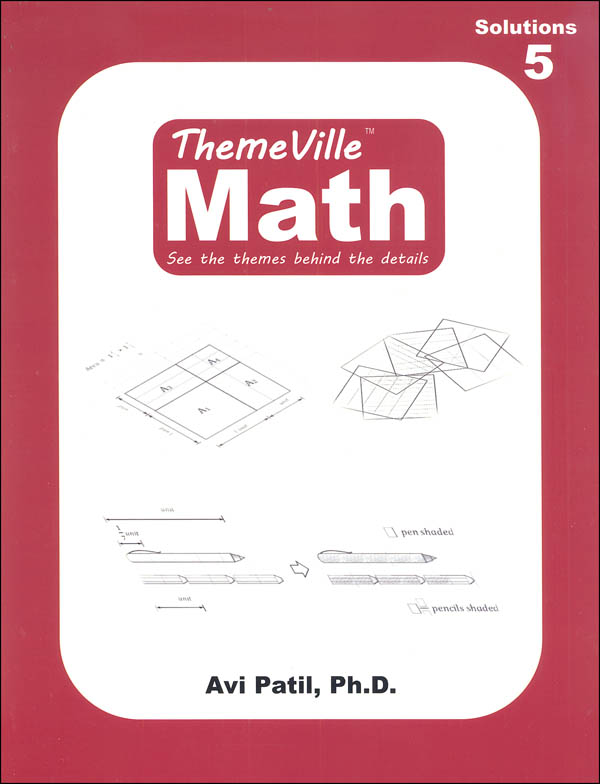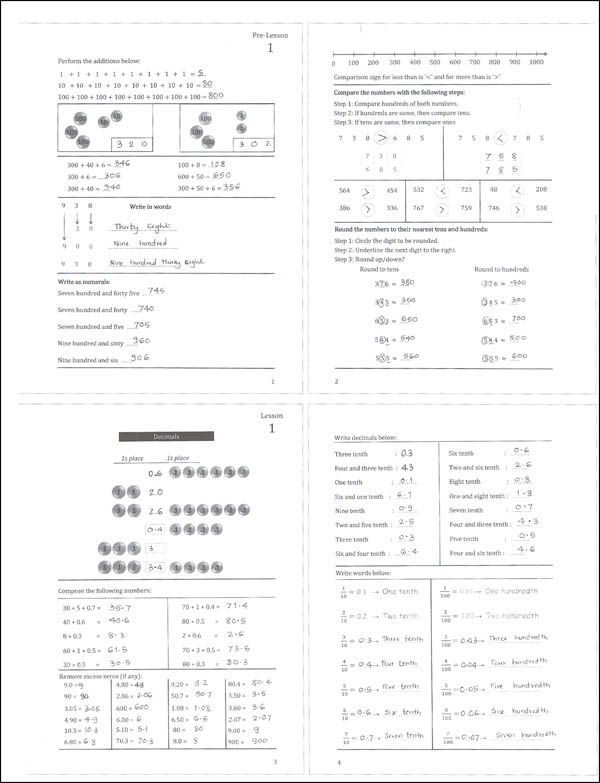••# ThemeVille Math Solutions 5

# 049495

Our Price: \$14.75
Retail: \$14.85
Save: 0.67% (\$0.10)
In Stock.
Qty:
Qty:

 Item #: 049495 9781641020459 5

#### Publisher Description:

This book has complete solutions to each problem for the level 5.

Category Description for Themeville Math Level 5:

Grade 5 includes review of the Levels 1-4 (the first fifteen lessons); multiplication/division with fractions; addition/subtraction with mixed numbers; decimals till millionths; finding the unknown in an expression; multiplication/division with decimals; area with fraction/decimal units; plotting graphs; units compatibility; word problems; exponents; combined operations; angles; volume.

Category Description for Themeville Math (Gr. 1-5):

Combine the daily review of Saxon, some of the methodology of a Singapore program, instructional videos, manipulatives to demonstrate lesson concepts, and you have the new program titled Themeville. I really like this straightforward program that combines desirable aspects of several different programs. This is titled Themeville because the lessons let you see the themes behind the details of the math you are learning.

There are four black & white student worktexts per level with over 1000 pages of instruction and practice. Written primarily for US homeschoolers instruction, word problems include US currency and common US names and city names. Comprehensive and easy to implement, it would be a good fit for many homeschool families. Each book is divided into daily lessons: 140+ per grade level. Each daily lesson follows the same format: pre-lesson, video/discussion, and lesson. The pre-lesson is a warm-up and reviews topics up to this point and includes, in many cases, simple math problems to keep math facts sharp. Video/discussion is a time to watch the video on the publisher’s website and discuss the new topic. The lesson is where the new concept is introduced and practiced through activities. There is no separate, expensive teacher edition for this course. All Parent/Teacher notes are found in a shaded box at the top of the lesson’s first page. You will find plenty of practice with math facts and word problems in this series. Tests are found directly in the student worktext – one every ten lessons.

Themeville Math does a good job of presenting concepts in an orderly manner that makes sense to children and works to build a progressively solid math foundation: concrete, to pictorial, to conceptual. The program incorporates methods like modeling using ten-frame, visual models, and continual review. The student worktext doesn’t include an abundance of written instruction (similarly to Kumon workbooks); the video lessons and manipulative instruction are intended to teach the concept. Video lessons are included with curriculum purchase: create a user ID and password at the publisher’s website and in 2 to 3 days you will be e-mailed access. These videos are an important part of every lesson. If you are unsure of where to begin in the series, a placement test available.

Grade 1 covers counting in groups of 10s, 5s and 1s; place values of 10s and 1s; addition/subtraction within 20; length measurement; operations with number line/colored rods; partitioning/comparison of numbers; money; chart of 0-100; numerals; skip counting; counting forward/backward; word problems; ordinal numbers.

Grade 2 includes review of the first ten lessons in Level 1; place values of 100s,10s and 1s; multiplication/division within 100; even & odd numbers; long addition/subtraction; operations with arrays; number line; money; numerals; comparison/rounding of numbers; plane / solid figures; perimeter; clock reading; calendar; graph reading/plotting; word problems.

Grade 3 includes review of the Levels 1-2 (the first fifteen lessons); long multiplication/division; long addition / subtraction, area and perimeter, symmetry, combined operations, number composition / decomposition with place values; words; comparison/rounding of numbers; clock/calendar reading and working with durations; data analysis and histogram; measurement units (kg – gm, lit – ml); word problems.

Grade 4 covers review of the Levels 1-3 (the first fifteen lessons); fractions; mixed numbers; addition/subtraction of fractions; factors; prime numbers; comparison/rounding of numbers; long division; decimals (tenths, hundredths, and addition/subtraction); big numbers; multiplication/division by 10 or 100; time calculations; word problems; area/perimeter.

Grade 5 includes review of the Levels 1-4 (the first fifteen lessons); multiplication/division with fractions; addition/subtraction with mixed numbers; decimals till millionths; finding the unknown in an expression; multiplication/division with decimals; area with fraction/decimal units; plotting graphs; units compatibility; word problems; exponents; combined operations; angles; volume.

Answer Keys for this series provide the complete solutions to all problems in the worktexts, including the pre-lesson exercises, lesson exercises, and tests. In cases of simpler problems that may not require an explanation (like math facts), there will just be answers. This book is small-format student pages with the answers/solutions.

Manipulative Kits are needed to demonstrate the concepts in the lessons. Kits are available for grades 1-3 and 4-5. The following items are included in the 1-3 kit: Play money set; colored rods (Cuisenaire® type), place value foam disks, place value strips for whole numbers and decimals; hundred number chart; area overlays (whole number multiplication); vinyl unit rulers 1-3; geared clock; plastic centimeter cubes; 12” ruler; 50 yards of ribbon; Rekenrek parts (Red/White beads and stems). Parents will need to assemble the Rekenrek and do a bit of prep with the unit rulers and place value strips. A manipulative preparation video on the publisher’s website will help with preparation.

The Full Manipulative Kit for Level 4-5 has the necessary manipulatives for levels 4 and 5 (use if you do not have the 1-3 kit manipulatives): play money set; place value foam disks; place value strips for whole numbers and decimals; vinyl unit rulers 4-5; area overlays – (for whole number multiplication/ Fractions / Mixed numbers multiplication / decimal multiplication); geared clock; centimeter plastic cubes ribbon (50 yd); 1 foot ruler; angle protractor.

If you already have the 1-3 kit, then you only need the Add-on kit for grades 4-5. It includes vinyl unit rulers 4-5; area overlays for fractions / mixed numbers multiplication / decimal multiplication; ribbon (50 yd); and angle protractor.

Although a relatively new program, this is a top pick for a solid and thorough approach to elementary math at an affordable price. Great for families on a budget who still want quality curriculum! ~ Donna

Category Description for COMPREHENSIVE PROGRAMS - ELEMENTARY:

Primary Subject
Mathematics
5
ISBN
9781641020459
Format
Paperback
Brand Name
Epistology Learning
Weight
1.35 (lbs.)
Dimensions
11.0" x 8.62" x 0.5"